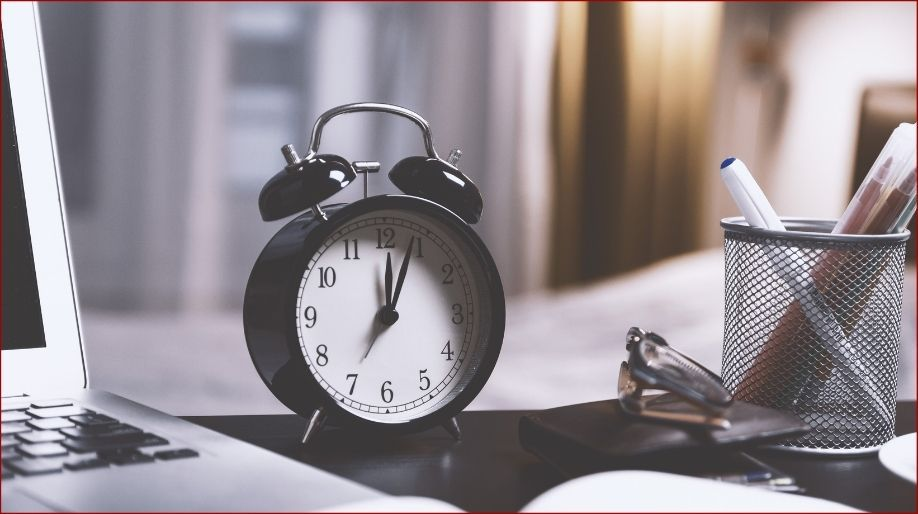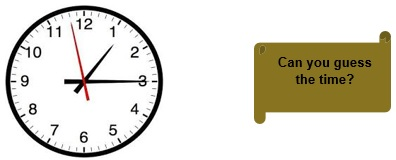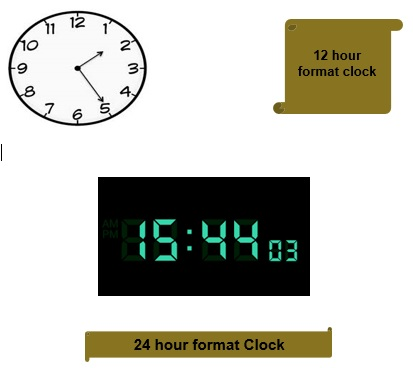Register for Maths, Science, English, Reasoning Olympiad Exams Register here | Book Free Demo for Live Olympiad Classes here | Check Olympiad Exam Dates here | Buy Practice Papers for IMO, IOM, HEO, IOEL etc here | Login here to participate in all India free mock test on every Saturday

# TimeHow do you answer when your parents ask “What is the time?" Surely we go and look at the clock and tell them the answer. Yes it’s pretty easy but let’s just understand the meaning of time and how to use and see time.• Time is an indefinite continued progress of events in the past, present and future. It simply means that each and every sequence of events which has happened, which is happening or which is going to happen the durations or intervals thus created is time.
• It is measured in hours, minutes and seconds.
• The instrument used to measure time accurately and more precisely is clock as shown in the above figure.

Understanding Clock

The clock is an instrument to measure time which is shown in hours, minutes and seconds.

Hour, Minute and Seconds are the units in which time is measured. For example 2 hour, 30 min and 12 seconds is the measurement of time at that moment.

The Hour hand is the smallest and slowest in clock, then comes Second hand and then the largest is Minute hand.

Time is represented as Hour: Minutes: Seconds

Example 1:- 04: 32:45

Before we proceed further let’s discuss and learn about some common things which we are using form very long time:

There are two types of clocks

• 12 hour Format clock: As the name suggests this clock shows time form 1 – 12. These are non-digital clocks. Example- the wall clocks.
• 24 hour Format clock:- As the name suggests this clock shows time form 1 – 24. These are digital clocks. Example – the clocks present on our smart phones.Whenever we tell someone time we tell it along with a suffix and that suffix is sometime A.M. and sometimes it’s P.M. But if I ask you how many of you know the full name and can define these two terms?

In a 12 hour clock the 24 hour time is divided into two periods 12 midnight to 12 noon and then 12 noon to 12 midnight and that’s how it covers all the 24 hours.

A.M.:- It stands for Ante Meridiem and it means before midday. Hence from 12 midnight to 12 noon we use A.M. suffix after the time. For example we say its 9 am.

P.M.:- It stands for Post Meridiem and it means past midday. Hence from 12 midday to 12 midnight we us P.M. suffix after time. For example we say its 4 pm.

Conversions

• 1 Hour = 60 Minutes
• 1 Minute = 1/60 Hour
• 1 Minute = 60 Seconds
• 1 Second = 1/60 Minutes

Example 2:- Convert 4 hour, 30 minutes into seconds.

Solution:- As we know that 1 hour = 60 minutes

Therefore 4 hours = 60 x 4 = 240 minutes.

And hence the total minutes = 240 + 30 = 270 minutes.

And as we know that 1 minute = 60 seconds.

Therefore 270 minutes = 270 x 60 = 16,200 seconds.

Let us learn how to convert 12 hour clock to a 24 hour clock in some basics steps:

• Count from 1 – 12 on a clock.
• After 12 begin from 1 and start writing 13 below it.
• Do the same for 2 and write 14 below it.
• Similarly below 3 write 15 and so on and finally below 12 write 24.
• Now after 12 noon the as the clock will strike 1 pm we will say it as 13 hour and similarly for 2 we will say 14 hours and so on.

Hence we can create a table as:

 12 hour Format 24 Hour Format 1 pm 13 hour 2 pm 14 hour 5 pm 17 hour 8 pm 20 hour 11 am 11 hour 9 am 9 hour

Here we can notice that if time is in A.M. there is no difference between 12 hour and a 24 hour format clock. The difference arises only if time is in P.M.

Here another important point arises that the difference is always 12 if we subtract the time in 24 hour clock to 12 hour clock. Look at the table carefully you will observe it.

Example 3:- Convert 9:45:12 P.M. in 24 hour format clock.

Solution:- While changing the clock format we only have to worry about the value of hour as rest values will remain same.

So in 24 hour format 9 means 9 + 12 = 21 hour.

Therefore the time in 24 hour format clock will be 21:45:12.

Example 4:- Repeat the above example if the time given is in A.M.

Solution:- As we know that if time is given in A.M. it is same for both 12 hour and 24 hour clock format.

Hence the time will be 9:45:12 in 24 hour format clock.

Practice Questions

Question 1:- How many minutes are there in 2 hours?

Question 2:- How many seconds are there in 45 minutes?

Question 3:- How many hours are there in 300 minutes?

Question 4:- How many hours are there in 7200 seconds?

Question 5:- State True or False:

1. There are two types of clocks
2. Digital clock shows 12 hour format only.
3. M. is used from 12 midday to 12 midnight.
4. 1 minute = 60 hours
5. 1 hour = 60 seconds.

Recap

• Time is a measurement of intervals of sequences.
• It is measured using an instrument called clock.
• A clock contains an hour, a minute and a second hand.
• There are two types of clocks only.
• M. stands for Ante Meridiem.
• M. stands for Post Meridiem.
• There is difference of 12 between every value of a 12 hour clock and a 24 hour clock if the time is in P.M.
• There is no difference if the time is in A.M.

## Quiz for Time

 Q.1 Which of the following month has 30 days? a) January b) February c) March d) April
 Q.2 How many big divisions are there in a clock dial? a) 5 b) 12 c) 6 d) 60
 Q.3 How many hours are there in the month of August? a) 744 b) 720 c) 24 d) 60
 Q.4 The time from 12 o' clock midnight to 12 o' clock noon is called ______ a) PM b) AM c) Before noon d) Both a and c
 Q.5 Small hand shows ______. a) Hours b) Minutes c) Both a and b d) None of the above
 Q.6 The number of days in an ordinary year is ______. a) 365 b) 366 c) 12 d) 24
 Q.7 How many minutes are there in between 4:00 PM and 7:30 PM? a) 180 b) 210 c) 3 d) 30
 Q.8 How many hours are there in 14 days? a) 14 b) 168 c) 840 d) 336
 Q.9 How many minutes does it take to complete one round of the clock? a) 12 b) 30 c) 60 d) 24
 Q.10 Radhika went to temple at 7:00 AM and came back at 8:30 AM. How much time did she spend in temple? a) 90 minutes b) 5400 seconds c) Both a and b d) 1 hour